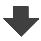Search:

# Examples of electrolysis

## Exercise 1

Electrolysis of a copper(II) bromide solution is done between (inert) graphite electrodes. Give the formulas of the ions which are in presence !

Ions: $Cu^{2+}$ ; $Br^{-}$ from dissolution and dissociation of copper(II) bromide $H^{+}$; $OH^{-}$ from the partial dissociation of water.

Look for the strongest oxidant - for the strongest reductant !

$Cu^{2+}$ is the strongest oxidant $Br^{-}$ is the strongest reductant.

Write the equations of the reactions occuring at the electrodes !

Cathode: $Cu^{2+}$ $+$ $2e^-$ $\longrightarrow$ $Cu$ (reduction) Anode: $2Br^{-}$ $-$ $2e^-$ $\longrightarrow$ $Br_2$ (oxidation)

What is observed?

At the cathode: deposit of metallic red copper on the electrode. At the anode: formation of brownish-orange bromine which dissolves in water.